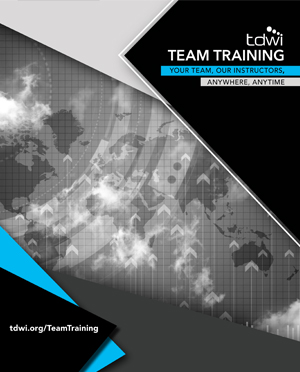## TEAM TRAINING

Our Instructors
Anywhere, Anytime

Duration: Three Day Workshop

Prerequisite: Attendees will need a laptop computer with specific software installed prior to the session. In advance of the class, they will receive detailed instructions for software download and installation.

Course Outline

This intensive three-day workshop covers what every business person needs to know to build their own analytic models.

Designed specifically for business users, students will learn to craft, train, and evaluate predictive models without requiring any background in statistics or programming. Students will learn some of the same techniques used by data scientists—including linear regression, logistic regression, and decision tree models. Concepts are reinforced through a series of hands-on exercises and templates using Microsoft Excel, and students will learn to apply their Excel skills in the open source machine learning tool R.

Attendees will learn to build basic predictive models using linear regression, a statistical technique that models the relationship between two data variables. This allows the student to answer diagnostic questions (why something happened) and build predictive solutions (what will happen.)  Students will learn what types of problems are best suited to linear regression and what kind of data is required. They will put these skills into practice through workshop exercises using Microsoft Excel. Excel’s Analysis ToolPak handles the math, so attendees focus on learning the concepts required to build, validate, and interpret linear regression models.

Next, students will learn to build advanced analytic models using logistic regression—a statistical technique that models available data to predict a specific outcome. Attendees will learn when logistic regression is appropriate, based on the business question and the structure of the data. They will practice building, validating, and interpreting logistic regression models using Excel, and will receive templates to craft their own models.

The final day of the program translates these skills into the world of machine learning using R. Through lessons designed specifically for people familiar with Excel, students will learn how to use the R environment for more robust models, including decision trees, random forest techniques, and more.

• How to rigorously identify trends/patterns in business data
• How to rigorously compare groups/collections of business data
• The types of business problems where linear regression can be useful
• Why the arithmetic mean (aka the average) is a predictive model
• How simple linear regression improves upon the arithmetic mean
• How to use Excel to train simple linear regression models
• Ways to use Excel to train multiple linear regression models
• How to interpret linear regression models in terms of business drivers
• Ways to evaluate the effectiveness of your linear regression models
• The types of business problems where logistic regression can be useful
• The basics of probabilities and odds ratios
• Templates that streamline logistic regression analyses in Excel
• How to use the Excel Solver to train simple logistic regression models
• How to use the Excel Solver to train multiple logistic regression models
• Ways to evaluate the effectiveness of your logistic regression models
• How to interpret logistic regression models in terms of business drivers
• Avoiding the “gotchas” of logistic regression models
• Machine learning to solve classification problems
• The decision tree algorithm, including pros/cons
• The mighty random forest algorithm and how it "fixes" decision trees
• How to test your random forest for accuracy
• Data analysis and feature engineering using random forests
• How to analyze your feature engineering efforts: did they work?

#### Geared To

• Managers
• Knowledge workers
• Executives
• Database developers
• BI and analytics developers
• Anyone who wants to move the needle on the business using data

#### REQUEST INFORMATIONYvonne M. Baho Director, Enterprise Learning
Phone: 978.582.7105
Email: ybaho@tdwi.org### REQUEST INFORMATIONYvonne M. Baho
Director, Enterprise Learning
Phone: 978.582.7105
E-mail: ybaho@tdwi.org